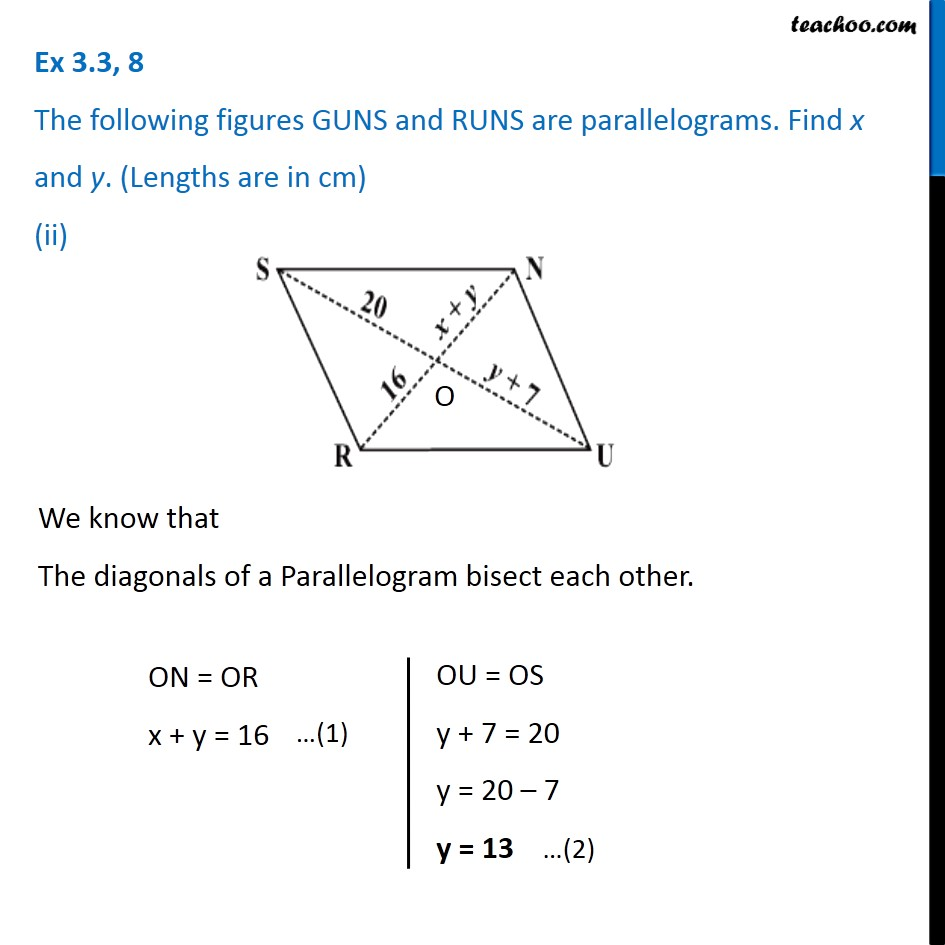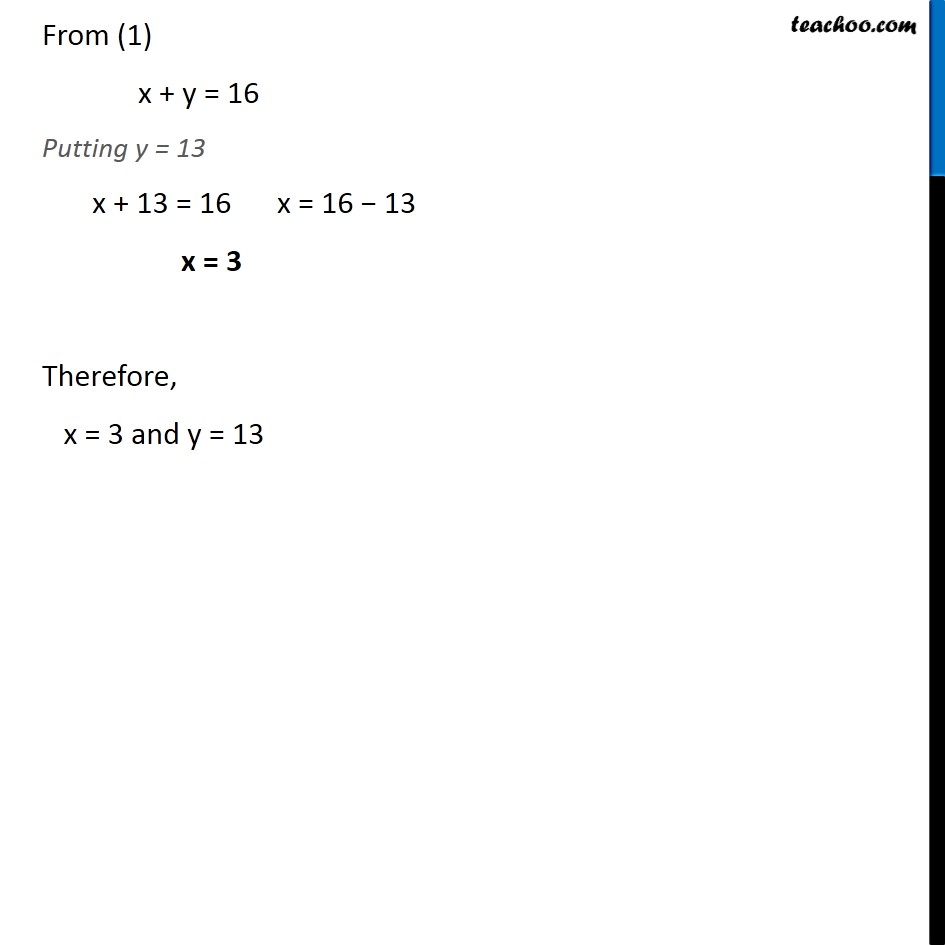Ex 3.3

Chapter 3 Class 8 Understanding Quadrilaterals
Serial order wise549 people joined Teachoo Black. What are you waiting for?

### Transcript

Ex 3.3, 8 The following figures GUNS and RUNS are parallelograms. Find x and y. (Lengths are in cm) (ii) We know that The diagonals of a Parallelogram bisect each other. ON = OR x + y = 16 OU = OS y + 7 = 20 y = 20 – 7 y = 13 From (1) x + y = 16 Putting y = 13 x + 13 = 16 x = 16 − 13 x = 3 Therefore, x = 3 and y = 13